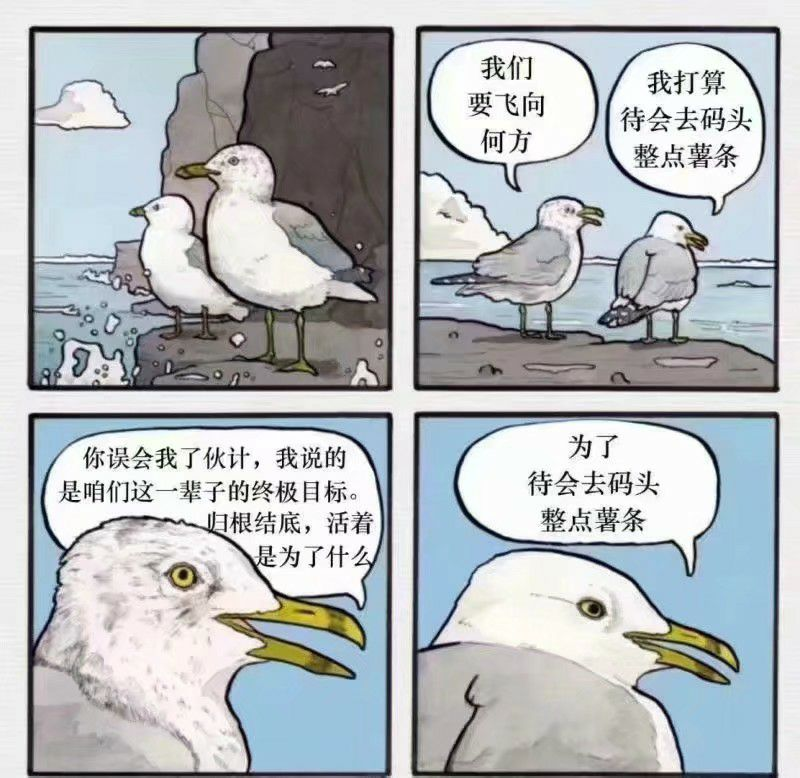2013-05-14

—— 我的一位朋友的青春疼痛（中二）日记

• 美丽值：$P_1 = x_1 = \frac{1}{n} \sum_{i=1}^n \frac{1}{5}a_i$，凭感觉打分
• 旁人值：若周围有路人，每有一人则减一万分

$$x_2 = 0, P_2 = 20$$
$$x_2 > 0, P_2 = -10000x_2$$

• 亮度值：$P_3=\frac{1}{5} {104 - 2(\frac{x_3}{20} + \frac{20}{x_3}) }$，简而言之，蜡烛的亮度最高，其他均逐渐降低
• 幽静值：说白了，就是如果噪音大，氛围值就会变成负无穷，$P_4 = 30 - \frac{1000}{t}$
• 沉默对视值：$P_5=\frac{1}{5}S$，简而言之对视时间（单位：秒）越长，分数也越高

$$30 \leq x_5 \leq 60, S = 100$$
$$60 < x_5, S = 100 - 5 (x_5-60)$$## 小镇很少踏足，却是瞭望时常常看到的小镇一角，也是建筑最多的一处

## 家## 我你越来越像小圭了估计会长成一个无聊的大人

——「天气之子」影评## 学生Q.E.D.

Always To Be Continued.